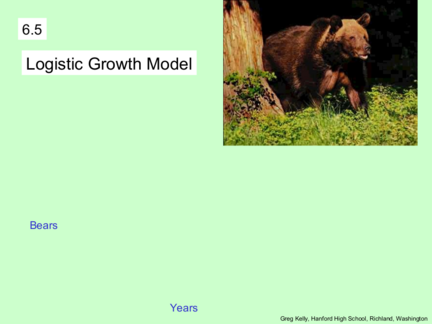# Logistic Growth Model of an Exponential FunctionContributed by:We will discuss here the logistic growth model of an exponential function. Also, some examples related to the logistic growth model.
1. Logistic Growth Model
Bears
Years
Greg Kelly, Hanford High School, Richland, Washington
2. kt
We have used the exponential growth equation y  y0 e
to represent population growth.
The exponential growth equation occurs when the rate of
growth is proportional to the amount present.
If we use P to represent the population, the differential
equation becomes: dP
kP
dt
The constant k is called the relative growth rate.
dP / dt
k
P 
3. kt
The population growth model becomes: P  P0 e
However, real-life populations do not increase forever.
There is some limiting factor such as food, living space or
waste disposal.
There is a maximum population, or carrying capacity, M.
A more realistic model is the logistic growth model where
growth rate is proportional to both the amount present (P)
and the fraction of the carrying capacity that remains: M  P
M

4. The equation then becomes:
dP M  P
kP  
dt  M 
Our book writes it this way:
Logistics Differential Equation
dP k
 P  M  P
dt M
We can solve this differential equation to find the logistics
growth model.

5. Logistics Differential Equation
dP k
 P  M  P
dt M 1 A B
 
1 k P  M  P P M  P
dP  dt
P  M  P M 1  A  M  P   BP Partial
Fractions
1 1 1  k 1  AM  AP  BP
   dP  dt
M  P M  P M
1  AM 0  AP  BP
1 AP BP
ln P  ln  M  P  kt  C A A B
M
P 1
ln kt  C B
M P M 
6. Logistics Differential Equation
dP k
 P  M  P P
dt M e kt C
M P
1 k
dP  dt
P  M  P M M P
e  kt  C
P
1 1 1  k
   dP  dt M
M  P M  P M  1 e  kt  C
P
ln P  ln  M  P  kt  C M
1  e  kt  C
P
P
ln kt  C
M P 
7. Logistics Differential Equation
P kt C M
e P
M P 1  e  kt  C
M P M
e  kt  C P
P 1  e  C e  kt
M C
 1 e  kt  C Let A e
P
M M
1  e  kt  C P
P 1  Ae  kt

8. Logistics Growth Model
M
P
1  Ae  kt

9. Logistic Growth Model
Ten grizzly bears were introduced to a national park 10
years ago. There are 23 bears in the park at the present
time. The park can support a maximum of 100 bears.
Assuming a logistic growth model, when will the bear
population reach 50? 75? 100?

10. Ten grizzly bears were introduced to a national park 10
years ago. There are 23 bears in the park at the present
time. The park can support a maximum of 100 bears.
Assuming a logistic growth model, when will the bear
population reach 50? 75? 100?
M
P M 100 P0 10 P10 23
1  Ae  kt

11. M
P M 100 P0 10 P10 23
1  Ae  kt
100
10  At time zero, the population is 10.
1  Ae0
100
10 
1 A
10  10 A 100
10 A 90
100
A 9 P
1  9e  kt

12. M
P M 100 P0 10 P10 23
1  Ae  kt
100
P
1  9e  kt
100
23  After 10 years, the population is 23.
1  9e  k 10
 10 k 100  10k  0.988913
1  9e 
23
k 0.098891
 10 k 77
9e 
23
 10 k 100
e 0.371981 P
1  9e  0.1t 
13. 100
1  9e  0.1t
We can graph Bears
this equation
and use “trace”
to find the
Years
y=50 at 22 years
y=75 at 33 years
y=100 at 75 years